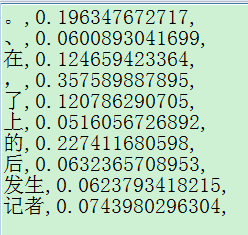Python怎么将列表，或者txt文件输出转化为字典啊？就是要将这样的数据转化为一一对应的字典？怎么做啊？我本来是用
coslist1.append(lines0)
coslist2.append(line0)
print (type(coslist2))
cosdic1=dict(coslist1)
cosdic2=dict(coslist2)
print(type(cosdic1))但是说列表转化不了字典？那我该怎么转化啊？大神们帮菜鸟出出主意

3个回答

• Code in python 3
``````rate1 = open('1.txt', 'r', encoding='utf-8')
dic = dict()
for line in rate1:
line = line.strip().split(',')
dic[line] = line
print(dic)
rate1.close()
``````